## ATtiny85 Graphics Display

27th April 2017

This article describes a 64x48 monochrome OLED display based on an ATtiny85. I've included three sample applications: a simple oscilloscope, a wireframe animation, and an analogue voltmeter: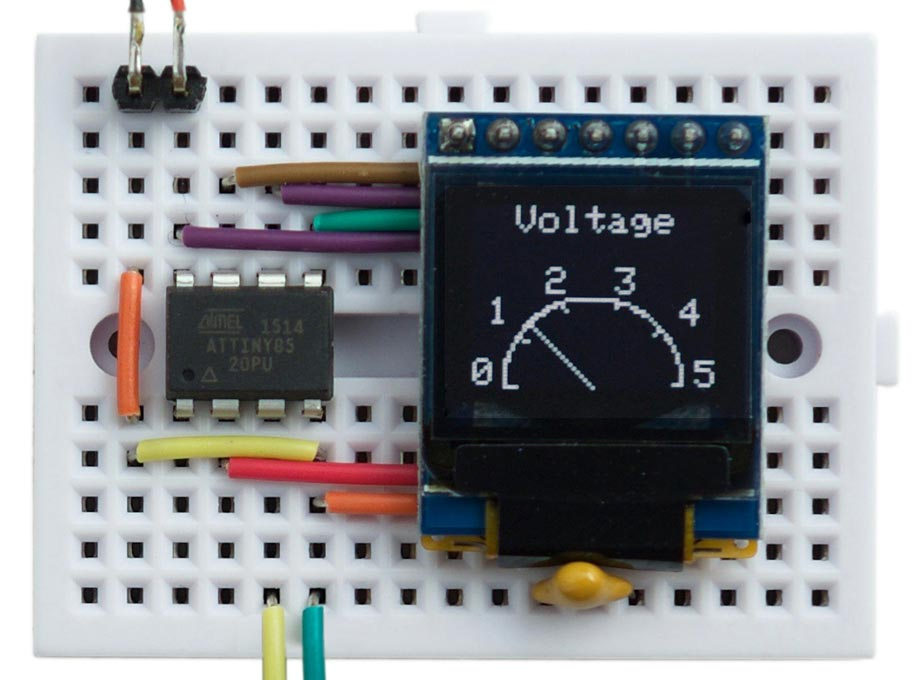Analogue voltmeter using a 64x48 OLED graphics display based on an ATtiny85.

It should be relatively straightforward to redesign the code to work with any other AVR processor.

Update: For an extension to this program that adds the ability to plot double-size characters see Big Text for Little Display.

### Introduction

I recently wanted a flexible way of displaying information from a project, and found this small monochrome 64x48 OLED display on Aliexpress . A similar one is available from Sparkfun . Although both SPI and I2C versions are available, I chose the SPI version because the display update is faster.

The display needs 4 pins to drive it, just within the capabilities of the ATtiny85 and leaving one pin available for another application. You can't read the display memory, so to do graphics you need to write into a buffer in RAM, and then copy this to the display. Because the display is 64x48 pixels it requires 64x48/8 or 384 bytes of memory for the graphics buffer, again just within the capabilities of the ATtiny85.

Here's the circuit: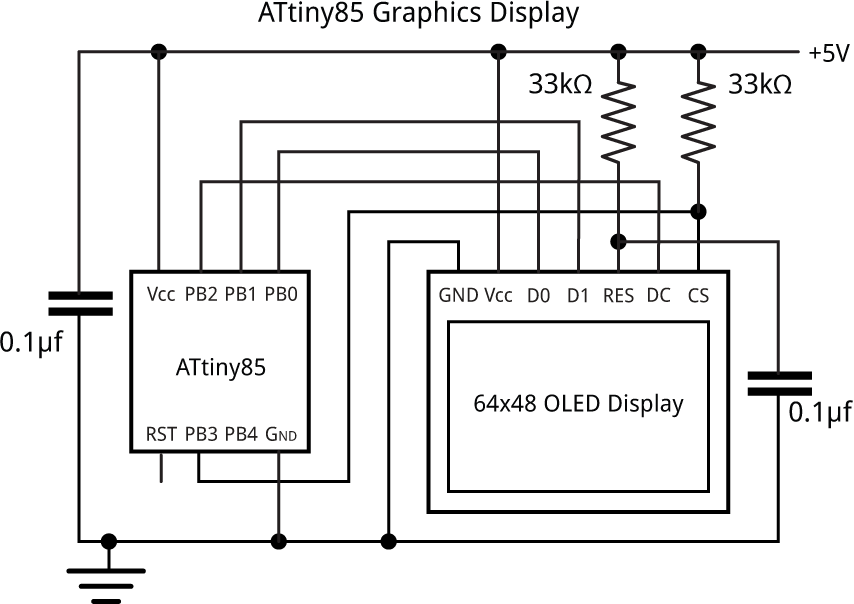Circuit of the 64x48 OLED graphics display based on an ATtiny85.

The resistor from the display's CS pin holds the chip select high to prevent the display from being affected by the ISP signals while programming the ATtiny85.

### Driving the display

The display uses the SSD1306 driver chip  which divides up the display into columns one pixel wide, and horizontal bands eight pixels high which are referred to as pages.

The graphics commands to plot points, draw lines, and draw text, all edit a buffer, which stores one bit for each pixel on the display. This is defined as follows:

```// Screen buffer
const int Buffersize = 64*6;
unsigned char Buffer[Buffersize];```

The DisplayBuffer() routine display the contents of the buffer by copying the bytes to the display. For this application we're using the display's Horizontal Addressing mode, which copies bytes to the display memory from left to right and top to bottom. To use this mode you just specify the column and page ranges with the commands 0x21 and 0x22, and then send the 384 bytes.

The SSD1306 is designed to handle displays up to 128x64, and the 64x48 display is positioned in the centre of this area, so to address it you need to select columns 32 to 95 (inclusive) and pages 2 to 7 (inclusive):

```void DisplayBuffer() {
PINB = 1<<cs; // cs low
Command(0x21); Command(32); Command(95);
Command(0x22); Command(2); Command(7);
for (int i = 0 ; i < Buffersize; i++) Data(Buffer[i]);
PINB = 1<<cs; // cs high
}```

This calls Data() which writes a byte to the display:

```void Data(uint8_t d) {
uint8_t changes = d ^ (d>>1);
PORTB = PORTB & ~(1<<data);
for (uint8_t bit = 0x80; bit; bit >>= 1) {
PINB = 1<<clk; // clk low
if (changes & bit) PINB = 1<<data;
PINB = 1<<clk; // clk high
}
}```

I spent a bit of time optimising the Data() routine, to make the display update as fast as possible. This version uses a variable changes to determine whether the data pin, PB1, should be changed for each bit to be output. This allows us to write to the PINB port to toggle the bit. With an 8 MHz ATtiny85 this routine updates the display in about 5.4 msec. This implies that we can update the display about 180 times a second, fast enough for simple animations.

Commands are identified by taking the dc pin low:

```void Command(uint8_t c) {
PINB = 1<<dc; // dc low
Data(c);
PINB = 1<<dc; // dc high
}```

Finally, InitDisplay() reads the display setup parameters from program memory, and writes them to the display:

```void InitDisplay () {
PINB = 1<<cs; // cs low
for (uint8_t c=0; c<InitLen; c++) Command(pgm_read_byte(&Init[c]));
PINB = 1<<cs; // cs high
}```

### Plotting points

I wrote some basic graphics routines for plotting points and drawing lines. These work on a conventional coordinate system with the origin at lower left: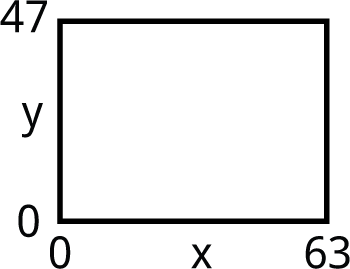You can move the origin by changing xOrigin and yOrigin; for example, to have the origin in the centre do:

`xOrigin = 32; yOrigin = 24;`

First the routine that plots a point:

```void PlotPoint(int x, int y) {
int row = 47 - y - yOrigin;
int col = x + xOrigin;
int page = row>>3;
int bit = row & 0x07;
// Set correct bit in slice buffer
Buffer[page*64 + col] |= 1<<bit;
}```

Note that this doesn't do any checking, so either make sure you don't plot points outside the display area, or add a line to check the x and y values.

### Drawing lines

The line plotting is performed by the DrawTo() line-drawing routine, which uses Bresenham's line algorithm to draw the best line between two points without needing any divisions or multiplications :

```void DrawTo(int x1, int y1) {
int sx, sy, e2, err;
int dx = abs(x1 - x0);
int dy = abs(y1 - y0);
if (x0 < x1) sx = 1; else sx = -1;
if (y0 < y1) sy = 1; else sy = -1;
err = dx - dy;
for (;;) {
PlotPoint(x0, y0);
if (x0==x1 && y0==y1) return;
e2 = err<<1;
if (e2 > -dy) {
err = err - dy;
x0 = x0 + sx;
}
if (e2 < dx) {
err = err + dx;
y0 = y0 + sy;
}
}
}```

### Drawing text

For simplicity the routine to draw text ignores the bottom three bits of the y coordinate, and plots the characters in a single page. The routine accesses the character set from program memory. An abbreviated version of the character map is as follows:

```const uint8_t CharMap PROGMEM = {
{ 0x00, 0x00, 0x00, 0x00, 0x00, 0x00 },
{ 0x00, 0x00, 0x5F, 0x00, 0x00, 0x00 },
...
{ 0xFF, 0xFF, 0xFF, 0xFF, 0xFF, 0x00 }
};```

The first row defines the bit pattern for ASCII character 32, space, and so on up to character 127.

Because of the limited RAM available on the ATtiny85, the text to be plotted is also specified in program memory, and the PlotText() routine reads the characters using pgm_read_byte():

```void PlotText(int x, int y, PGM_P s) {
int p = (int)s;
int page = (47 - y - yOrigin)>>3;
while (1) {
if (c == 0) return;
for (uint8_t col = 0 ; col < 6; col++) {
Buffer[page*64 + x + xOrigin] = pgm_read_byte(&CharMap[c-32][col]);
x++;
}
}
}```

To define the text to be plotted as being in program memory use the PSTR() macro (for PROGMEM string). For example, to plot "ATtiny85" centred on the second line of the display, write:

`PlotText(6, 16, PSTR("ATtiny85"));`

### Applications

#### Analogue voltmeter

The first application is an analogue voltmeter that reads the voltage on the analogue input A2 (PB4), and displays it as a pointer on an analogue display:

```void AnalogueMeter () {
xOrigin = 32; yOrigin = 0;
const int Delta2 = 16;
int x = -(23<<9), y = 0;
ClearBuffer();
PlotText(-20, 40, PSTR("Voltage"));
for (int i = 0; i<=100; i++) {
if (i%20 == 0) {
MoveTo(x>>9, (y>>9));
DrawTo((x>>9) - (x>>12), (y>>9) - (y>>12));
}
if (i == (analogRead(A2)*25 + 25)>>8) {
MoveTo(x>>9, y>>9);
DrawTo(0, 0);
}
MoveTo(x>>9, y>>9);
x = x + ((y>>9) * Delta2);
y = y - ((x>>9) * Delta2);
if (i != 100) DrawTo(x>>9, y>>9);
}
PlotText(-31, 0, PSTR("0")); PlotText(27, 0, PSTR("5"));
PlotText(-27, 16, PSTR("1")); PlotText(23, 16, PSTR("4"));
PlotText(-12, 24, PSTR("2  3"));
DisplayBuffer();
}```

#### Simple oscilloscope

The second application reads the analogue signal on the analogue input A2 (PB4) and displays it as a waveform on the display.

For example, here it is displaying the triangular waveform from a waveform generator kit I bought on Banggood :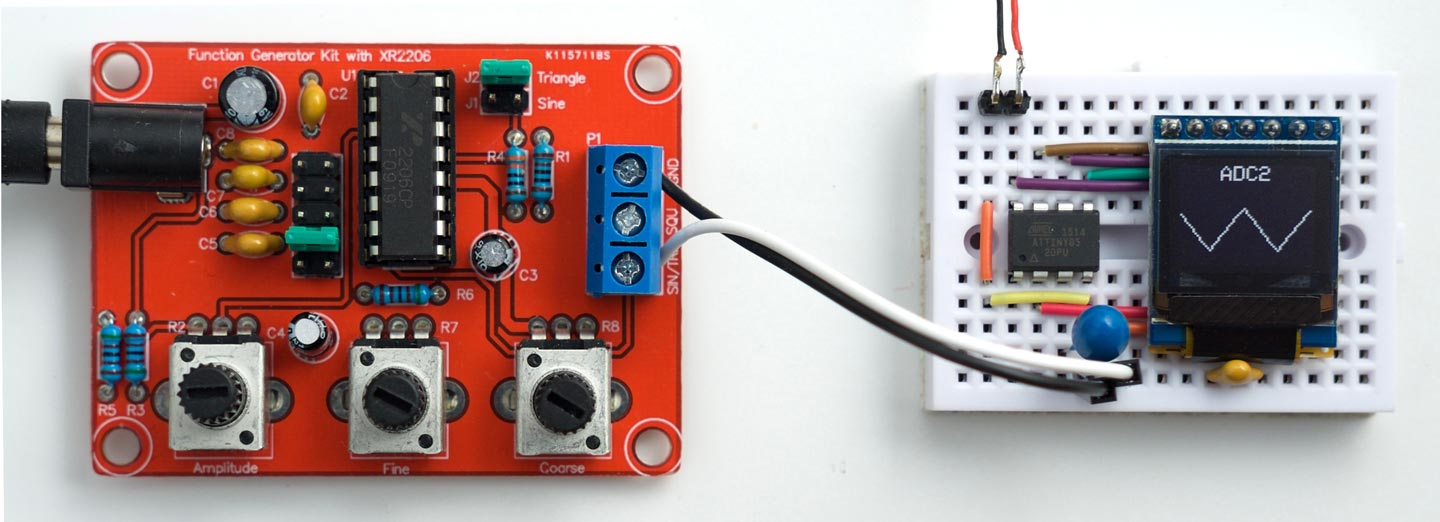Simple oscilloscope displaying a triangle wave, using the ATtiny85-based graphics display.

Here's the program:

```void Oscilloscope () {
xOrigin = 0; yOrigin = 0;
ClearBuffer();
for (int x=1; x<63; x++) {
if (x == 1) MoveTo(x, y); else DrawTo(x, y);
}
DisplayBuffer();
}
```

#### Animated cube

The final application generates an animated rotating wireframe cube: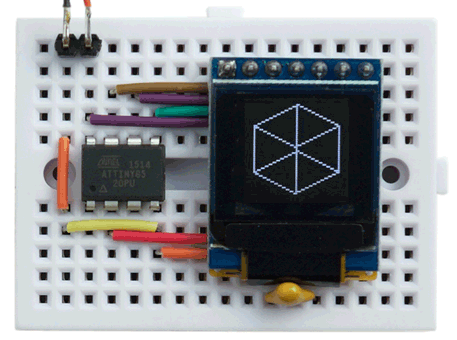Animated wireframe cube using the ATtiny85-based graphics display.

Here's the program:

```void RotatingCube () {
const int Delta = 9; // Approximation to 1 degree in radians * 2^9
xOrigin = 32; yOrigin = 24;
int x = 0, y = 22<<9;
for (;;) {
ClearBuffer();
int x9 = x>>9, y9 = y>>9, x10 = x>>10, y10 = y>>10;
// Top
MoveTo(x9, y10 + 12); DrawTo(y9, -x10 + 12);
DrawTo(-x9, -y10 + 12); DrawTo(-y9, x10 + 12);
DrawTo(x9, y10 + 12); DrawTo(x9, y10 - 12);
// Bottom
DrawTo(y9, -x10 - 12); DrawTo(-x9, -y10 - 12);
DrawTo(-y9, x10 - 12); DrawTo(x9, y10 - 12);
// Sides
MoveTo(y9, -x10 + 12); DrawTo(y9, -x10 - 12);
MoveTo(-x9, -y10 + 12); DrawTo(-x9, -y10 - 12);
MoveTo(-y9, x10 + 12); DrawTo(-y9, x10 - 12);
// Rotate cube
x = x + (y9 * Delta);
y = y - ((x>>9) * Delta);
DisplayBuffer();
}
}```

To run any of the examples put the appropriate call inside loop(); for example:

```void loop () {
RotatingCube();
}```

Here's the whole ATtiny85 Graphics Display program with the examples: ATtiny85 Graphics Display Program.

### Update

8th May 2018: Changed the declaration of the character map from uint32_t to uint8_t. Thanks to Larry Bank for pointing this out.

3rd December 2018: Fixed the circuit diagram so the CS pin is held high by the 33kΩ resistor (not low), and tidied up the program to make it consistent with Big Text for Little Display.

1. ^ White 0.66 inch OLED Display Module 64x48 from e_goto Processors on Aliexpress.
2. ^ SparkFun Micro OLED Breakout on Sparkfun.
3. ^ SSD1306 Datasheet on Adafruit.
4. ^ Bresenham's line algorithm on Wikipedia.
5. ^ Geekcreit XR2206 Function Signal Generator DIY Kit on Banggood.

Previous: Tiny Time 2 Watch